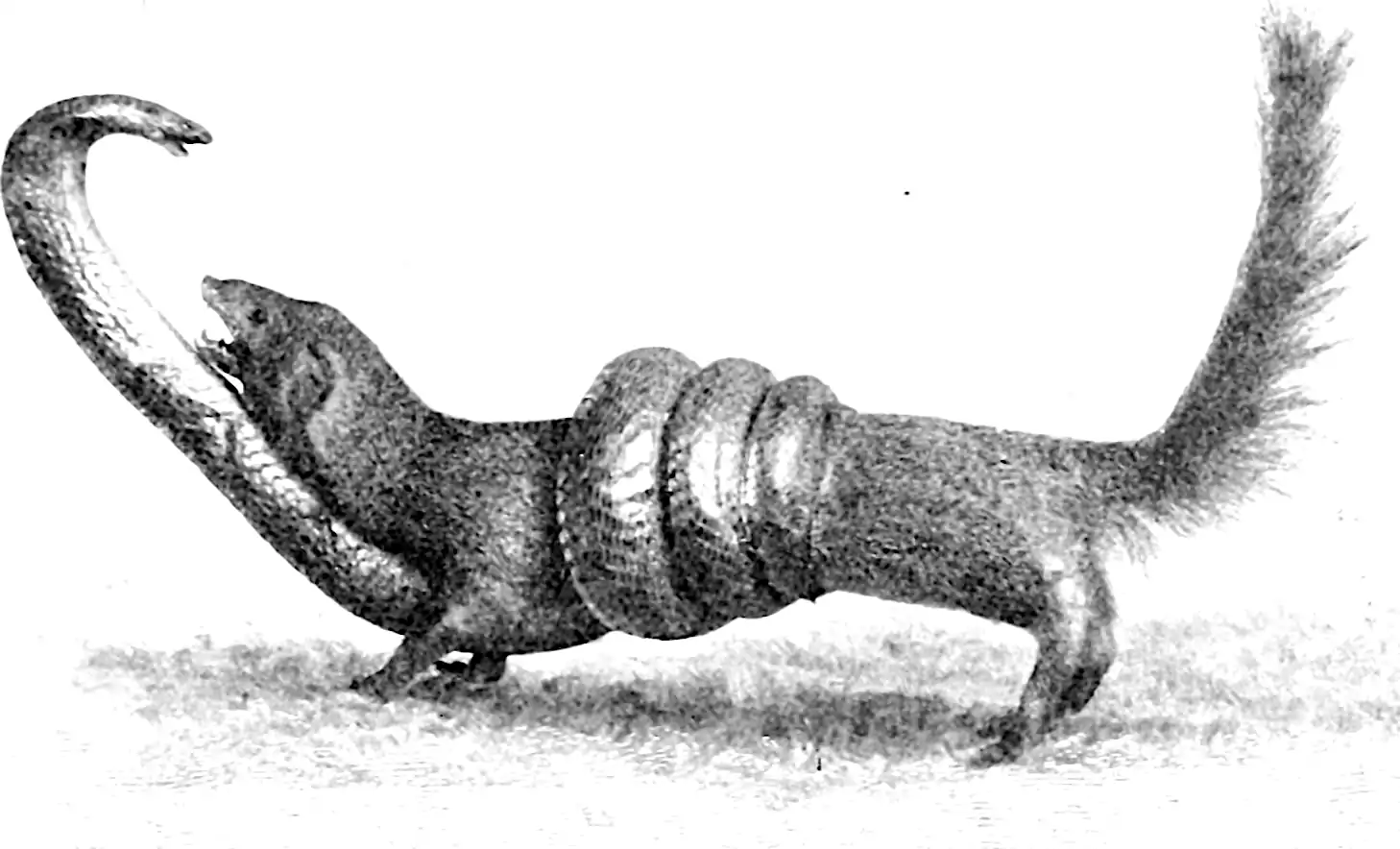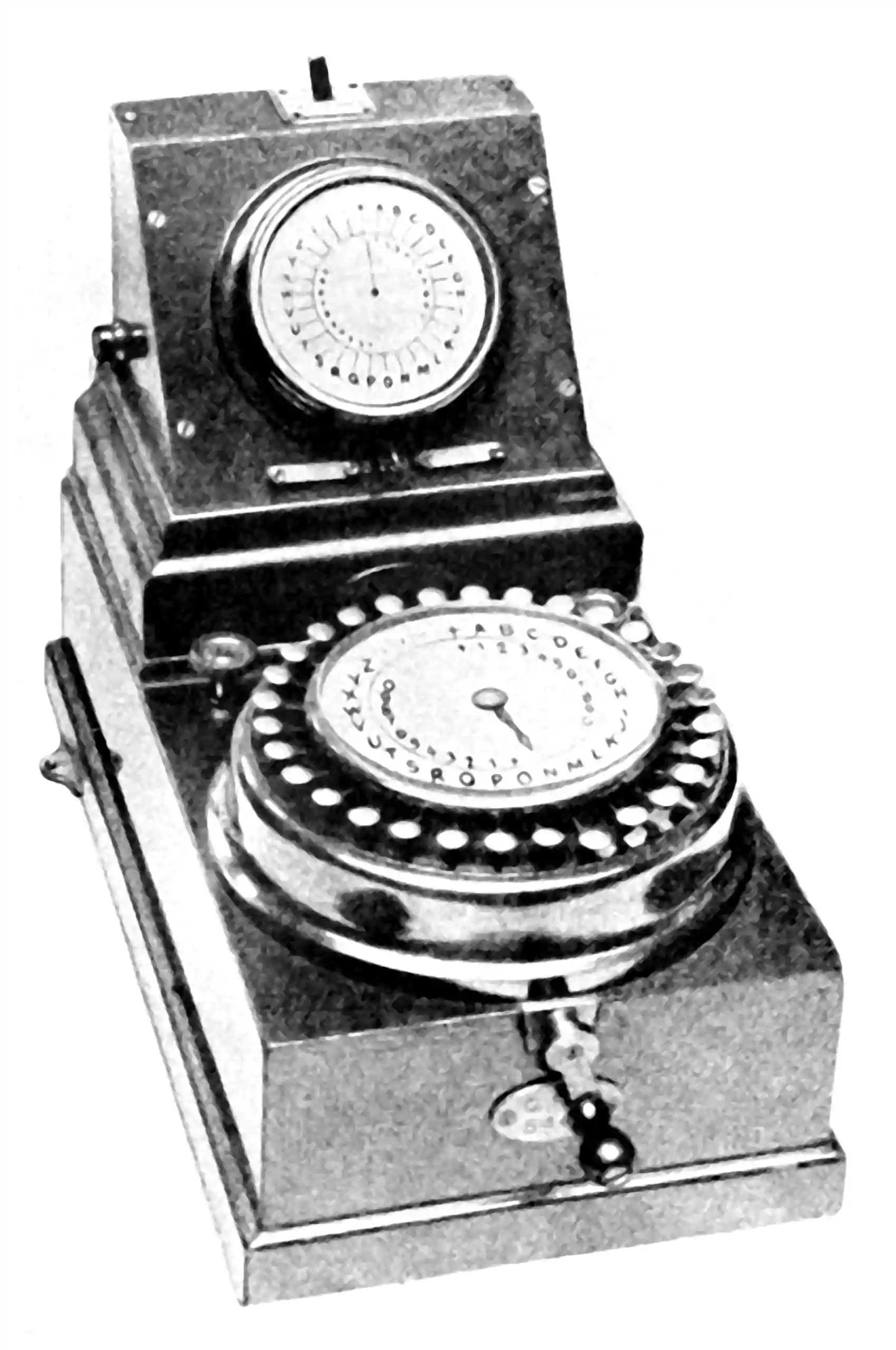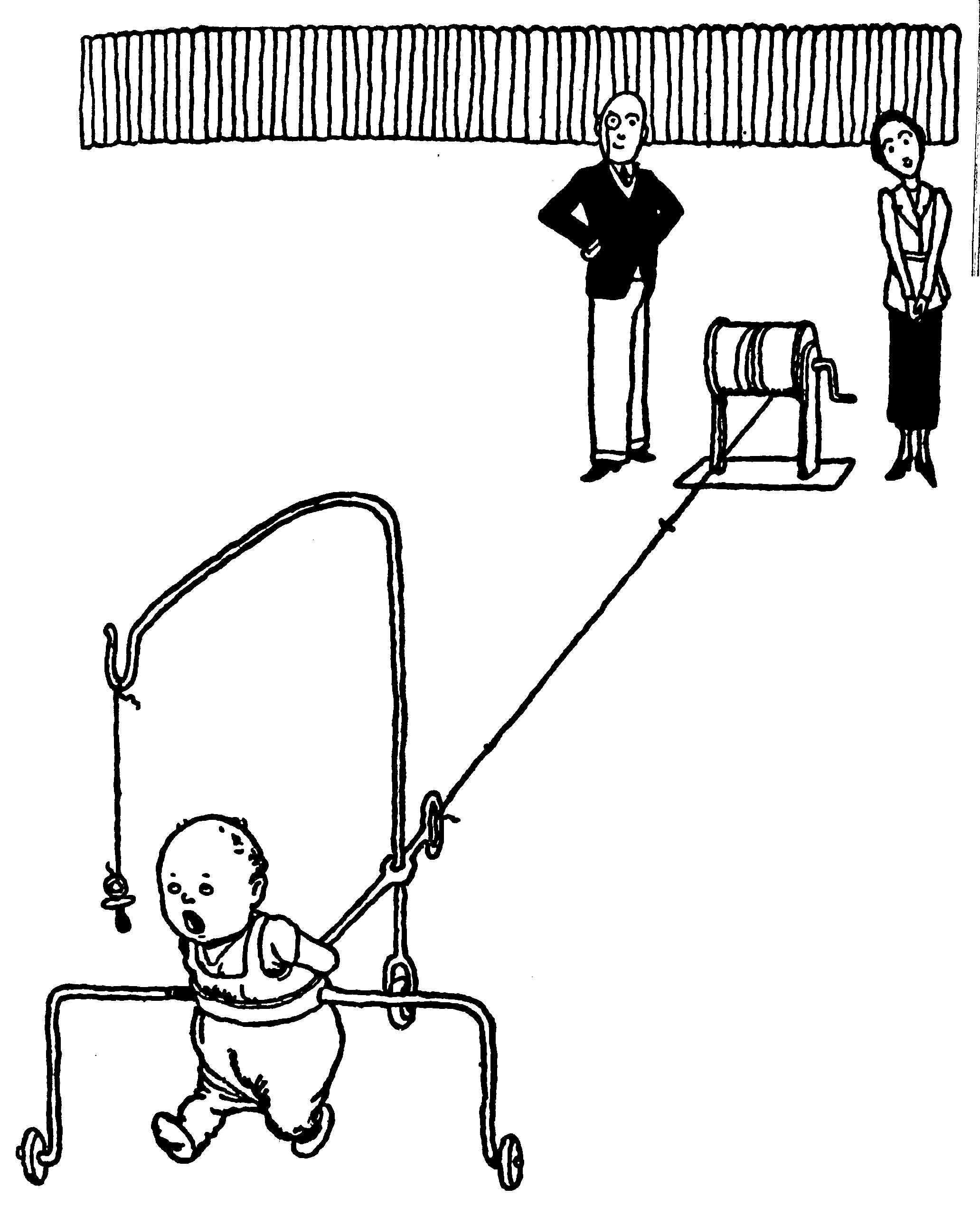# Predictive coding

## Does the model that our brains do bayesian variational prediction make any actual predictions about our brains?Maybe related (?) prediction processes. To learn: Is this what the information-dynamics folks are wondering about also, e.g. Ay et al. (2008) or Tishby and Polani (2011)? Perhaps this overvew of different brain models will place it in context: Neural Annealing: Toward a Neural Theory of Everything.

There is some interesting hype in this area, along the lines of understanding biological learning as machine learning: Predictive Coding has been Unified with Backpropagation, concerning Millidge, Tschantz, and Buckley (2020b). I have not read the article or the explanation properly, but at first glance it indicates that perhaps I do not understand this area properly. The assertion, skim-read, seems to be that predictive coding, which I imagined was some form of variational inference, can approximate minimum loss learning by backpropagation in some sense. While not precisely trivial, this would seem like well-trodden ground— unless I have failed ot understand how they are using the terms, which seems likely. TBC.

If we think there are multiple learning algorithms we find ourselves in a multi agent self situation.

## Layperson intros## “Free energy principle”

This section is dedicated to vivisecting a confusing discussion happening in the literature which I have not looked in to. It is could be a profound insight, or terminological confusion, or a re-statement of the mind-as-statistical learner idea with weirder prose style. I may return one day and decide which.

In this realm, the “free energy principle” is instrumental as a unifying concept for learning systems such as brains.

Here is the most compact version I could find:

The free energy principle (FEP) claims that self-organization in biological agents is driven by variational free energy (FE) minimization in a generative probabilistic model of the agent’s environment.

The chief pusher of this wheelbarrow appears to be Karl Friston (e.g. Friston 2010, 2013; Williams 2020). He starts his Nature Reviews Neuroscience with this statement of the principle:

The free-energy principle says that any self-organizing system that is at equilibrium with its environment must minimize its free energy.

Is that “must” in the sense that it is a

• moral obligation, or
• a testable conservation law of some kind?

If the latter, self-organising in what sense? What type of equilibrium? For which definition of the free energy? What is our chief experimental evidence for this hypothesis?

I think it means that any right thinking brain, seeking to avoid the vice of slothful and decadent perception after the manner of foreigners and compulsive masturbators, would do well to seek to maximise its free energy before partaking of a stimulating and refreshing physical recreation, such as a game of cricket. We do get a definition of free energy itself, with a diagram, which

…shows the dependencies among the quantities that define free energy. These include the internal states of the brain $$\mu(t)$$ and quantities describing its exchange with the environment: sensory signals (and their motion) $$\bar{s}(t) = [s,s',s''…]^T$$ plus action $$a(t)$$. The environment is described by equations of motion, which specify the trajectory of its hidden states. The causes $$\vartheta \supset {\bar{x}, \theta, \gamma }$$ of sensory input comprise hidden states $$\bar{x} (t),$$ parameters $$\theta$$, and precisions $$\gamma$$ controlling the amplitude of the random fluctuations $$\bar{z}(t)$$ and $$\bar{w}(t)$$. Internal brain states and action minimize free energy $$F(\bar{s}, \mu)$$, which is a function of sensory input and a probabilistic representation $$q(\vartheta|\mu)$$ of its causes. This representation is called the recognition density and is encoded by internal states $$\mu$$.

The free energy depends on two probability densities: the recognition density $$q(\vartheta|\mu)$$ and one that generates sensory samples and their causes, $$p(\bar{s},\vartheta|m)$$. The latter represents a probabilistic generative model (denoted by $$m$$), the form of which is entailed by the agent or brain…

$F = -<\ln p(\bar{s},\vartheta|m)>_q + -<\ln q(\vartheta|\mu)>_q$

This is (minus the actions) the variational free energy in Bayesian inference.

OK, so self-organising systems must improve their variational approximations to posterior beliefs? What is the contentful prediction?

See also: the Slate Star Codex Friston dogpile, based on an exposition by Wolfgang Schwarz.## Actual predictions about minds arising from predictive coding models

Does predictive coding tell us anything about minds in practice? Here are a some things that look like they must relate.

### Dark room problem

Basically: why do anything at all? Why not just live by self-fulfilling prophecies that guarantee good predictions, but ensuring that essentially nothing happens that requires not-trivial predictions?

Question: is this a perspective on depression?

## Computable

ForneyLab is a variational message passing library for probabilistic graphical models with an eye to computing it in a biologically plausible way and building predictive coding models.

ForneyLab is designed with a focus on flexibility, extensibility and applicability to biologically plausible models for perception and decision making, such as the hierarchical Gaussian filter (HGF). With ForneyLab, the search for better models for perception and action can be accelerated

## Incoming

What does this say about classical therapy, e.g. Acceptance and commitment therapy.

### No comments yet. Why not leave one?

GitHub-flavored Markdown & a sane subset of HTML is supported.